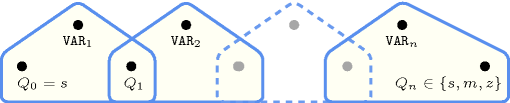## 5.168. global_contiguity

Origin
Constraint

$\mathrm{𝚐𝚕𝚘𝚋𝚊𝚕}_\mathrm{𝚌𝚘𝚗𝚝𝚒𝚐𝚞𝚒𝚝𝚢}\left(\mathrm{𝚅𝙰𝚁𝙸𝙰𝙱𝙻𝙴𝚂}\right)$

Synonym

$\mathrm{𝚌𝚘𝚗𝚝𝚒𝚐𝚞𝚒𝚝𝚢}$.

Argument
 $\mathrm{𝚅𝙰𝚁𝙸𝙰𝙱𝙻𝙴𝚂}$ $\mathrm{𝚌𝚘𝚕𝚕𝚎𝚌𝚝𝚒𝚘𝚗}\left(\mathrm{𝚟𝚊𝚛}-\mathrm{𝚍𝚟𝚊𝚛}\right)$
Restrictions
 $\mathrm{𝚛𝚎𝚚𝚞𝚒𝚛𝚎𝚍}$$\left(\mathrm{𝚅𝙰𝚁𝙸𝙰𝙱𝙻𝙴𝚂},\mathrm{𝚟𝚊𝚛}\right)$ $\mathrm{𝚅𝙰𝚁𝙸𝙰𝙱𝙻𝙴𝚂}.\mathrm{𝚟𝚊𝚛}\ge 0$ $\mathrm{𝚅𝙰𝚁𝙸𝙰𝙱𝙻𝙴𝚂}.\mathrm{𝚟𝚊𝚛}\le 1$
Purpose

Enforce all variables of the $\mathrm{𝚅𝙰𝚁𝙸𝙰𝙱𝙻𝙴𝚂}$ collection to be assigned value 0 or 1. In addition, all variables assigned to value 1 appear contiguously.

Example
$\left(〈0,1,1,0〉\right)$

The $\mathrm{𝚐𝚕𝚘𝚋𝚊𝚕}_\mathrm{𝚌𝚘𝚗𝚝𝚒𝚐𝚞𝚒𝚝𝚢}$ constraint holds since the sequence $0110$ contains no more than one group of contiguous 1.

All solutions

Figure 5.168.1 gives all solutions to the following non ground instance of the $\mathrm{𝚐𝚕𝚘𝚋𝚊𝚕}_\mathrm{𝚌𝚘𝚗𝚝𝚒𝚐𝚞𝚒𝚝𝚢}$ constraint: ${V}_{1}\in \left[0,1\right]$, ${V}_{2}\in \left[0,1\right]$, ${V}_{3}=1$, ${V}_{4}\in \left[0,1\right]$, $\mathrm{𝚐𝚕𝚘𝚋𝚊𝚕}_\mathrm{𝚌𝚘𝚗𝚝𝚒𝚐𝚞𝚒𝚝𝚢}$$\left(〈{V}_{1},{V}_{2},{V}_{3},{V}_{4}〉\right)$.

##### Figure 5.168.1. All solutions corresponding to the non ground example of the $\mathrm{𝚐𝚕𝚘𝚋𝚊𝚕}_\mathrm{𝚌𝚘𝚗𝚝𝚒𝚐𝚞𝚒𝚝𝚢}$ constraint of the All solutions slot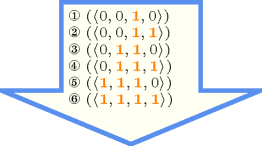Typical
 $|\mathrm{𝚅𝙰𝚁𝙸𝙰𝙱𝙻𝙴𝚂}|>2$ $\mathrm{𝚛𝚊𝚗𝚐𝚎}$$\left(\mathrm{𝚅𝙰𝚁𝙸𝙰𝙱𝙻𝙴𝚂}.\mathrm{𝚟𝚊𝚛}\right)>1$ $\mathrm{𝚊𝚝𝚕𝚎𝚊𝚜𝚝}$$\left(2,\mathrm{𝚅𝙰𝚁𝙸𝙰𝙱𝙻𝙴𝚂},1\right)$
Symmetry

Items of $\mathrm{𝚅𝙰𝚁𝙸𝙰𝙱𝙻𝙴𝚂}$ can be reversed.

Arg. properties

Contractible wrt. $\mathrm{𝚅𝙰𝚁𝙸𝙰𝙱𝙻𝙴𝚂}$.

Usage

The article [Maher02] introducing this constraint refers to hardware configuration problems.

Algorithm

A filtering algorithm for this constraint is described in [Maher02].

Counting

 Length ($n$) 2 3 4 5 6 7 8 9 10 11 12 13 14 15 16 17 18 19 20 21 22 23 24 Solutions 4 7 11 16 22 29 37 46 56 67 79 92 106 121 137 154 172 191 211 232 254 277 301

Number of solutions for $\mathrm{𝚐𝚕𝚘𝚋𝚊𝚕}_\mathrm{𝚌𝚘𝚗𝚝𝚒𝚐𝚞𝚒𝚝𝚢}$: domains $0..1$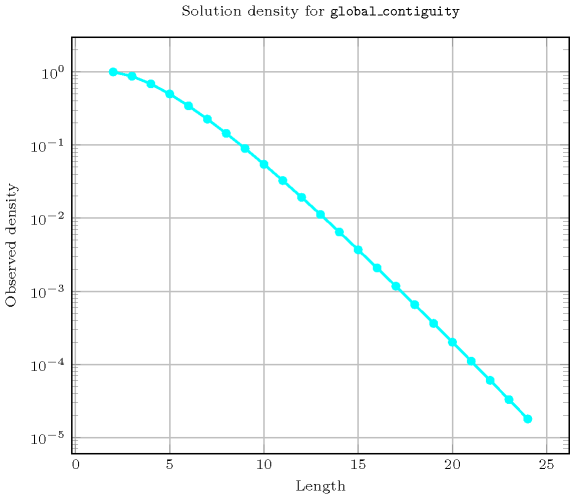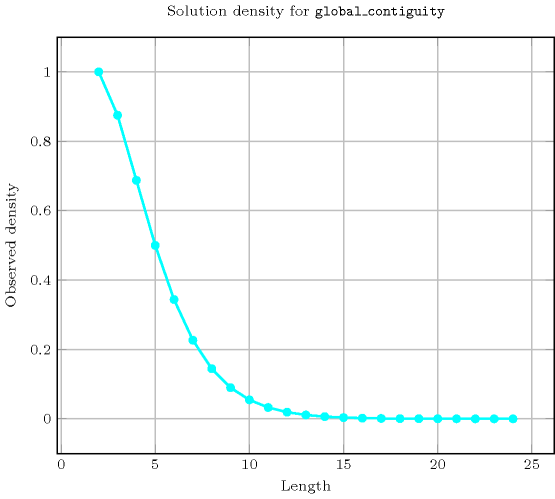Keywords
Cond. implications

$\mathrm{𝚐𝚕𝚘𝚋𝚊𝚕}_\mathrm{𝚌𝚘𝚗𝚝𝚒𝚐𝚞𝚒𝚝𝚢}\left(\mathrm{𝚅𝙰𝚁𝙸𝙰𝙱𝙻𝙴𝚂}\right)$

with  $|\mathrm{𝚅𝙰𝚁𝙸𝙰𝙱𝙻𝙴𝚂}|>2$

implies $\mathrm{𝚜𝚘𝚖𝚎}_\mathrm{𝚎𝚚𝚞𝚊𝚕}$$\left(\mathrm{𝚅𝙰𝚁𝙸𝙰𝙱𝙻𝙴𝚂}\right)$.

Arc input(s)

$\mathrm{𝚅𝙰𝚁𝙸𝙰𝙱𝙻𝙴𝚂}$

Arc generator
 $\mathrm{𝑃𝐴𝑇𝐻}$$↦\mathrm{𝚌𝚘𝚕𝚕𝚎𝚌𝚝𝚒𝚘𝚗}\left(\mathrm{𝚟𝚊𝚛𝚒𝚊𝚋𝚕𝚎𝚜}\mathtt{1},\mathrm{𝚟𝚊𝚛𝚒𝚊𝚋𝚕𝚎𝚜}\mathtt{2}\right)$ $\mathrm{𝐿𝑂𝑂𝑃}$$↦\mathrm{𝚌𝚘𝚕𝚕𝚎𝚌𝚝𝚒𝚘𝚗}\left(\mathrm{𝚟𝚊𝚛𝚒𝚊𝚋𝚕𝚎𝚜}\mathtt{1},\mathrm{𝚟𝚊𝚛𝚒𝚊𝚋𝚕𝚎𝚜}\mathtt{2}\right)$

Arc arity
Arc constraint(s)
 $•\mathrm{𝚟𝚊𝚛𝚒𝚊𝚋𝚕𝚎𝚜}\mathtt{1}.\mathrm{𝚟𝚊𝚛}=\mathrm{𝚟𝚊𝚛𝚒𝚊𝚋𝚕𝚎𝚜}\mathtt{2}.\mathrm{𝚟𝚊𝚛}$ $•\mathrm{𝚟𝚊𝚛𝚒𝚊𝚋𝚕𝚎𝚜}\mathtt{1}.\mathrm{𝚟𝚊𝚛}=1$
Graph property(ies)
$\mathrm{𝐍𝐂𝐂}$$\le 1$

Graph model

Each connected component of the final graph corresponds to one set of contiguous variables that all take value 1.

Parts (A) and (B) of Figure 5.168.2 respectively show the initial and final graph associated with the Example slot. The $\mathrm{𝚐𝚕𝚘𝚋𝚊𝚕}_\mathrm{𝚌𝚘𝚗𝚝𝚒𝚐𝚞𝚒𝚝𝚢}$ constraint holds since the final graph does not contain more than one connected component. This connected component corresponds to 2 contiguous variables that are both assigned to 1.

##### Figure 5.168.2. Initial and final graph of the $\mathrm{𝚐𝚕𝚘𝚋𝚊𝚕}_\mathrm{𝚌𝚘𝚗𝚝𝚒𝚐𝚞𝚒𝚝𝚢}$ constraint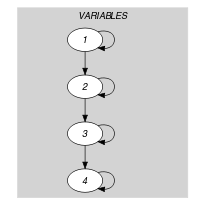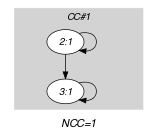(a) (b)
Automaton

Figure 5.168.3 depicts the automaton associated with the $\mathrm{𝚐𝚕𝚘𝚋𝚊𝚕}_\mathrm{𝚌𝚘𝚗𝚝𝚒𝚐𝚞𝚒𝚝𝚢}$ constraint. To each variable ${\mathrm{𝚅𝙰𝚁}}_{i}$ of the collection $\mathrm{𝚅𝙰𝚁𝙸𝙰𝙱𝙻𝙴𝚂}$ corresponds a signature variable that is equal to ${\mathrm{𝚅𝙰𝚁}}_{i}$. There is no signature constraint.

##### Figure 5.168.3. Automaton of the $\mathrm{𝚐𝚕𝚘𝚋𝚊𝚕}_\mathrm{𝚌𝚘𝚗𝚝𝚒𝚐𝚞𝚒𝚝𝚢}$ constraint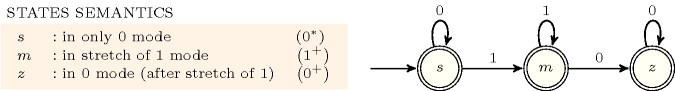##### Figure 5.168.4. Hypergraph of the reformulation corresponding to the automaton of the $\mathrm{𝚐𝚕𝚘𝚋𝚊𝚕}_\mathrm{𝚌𝚘𝚗𝚝𝚒𝚐𝚞𝚒𝚝𝚢}$ constraint